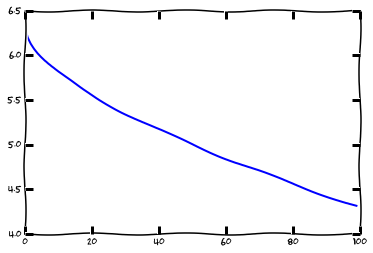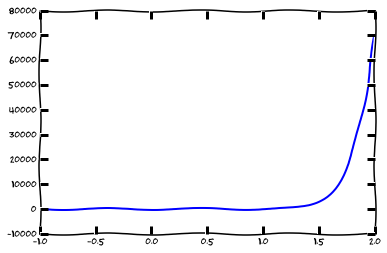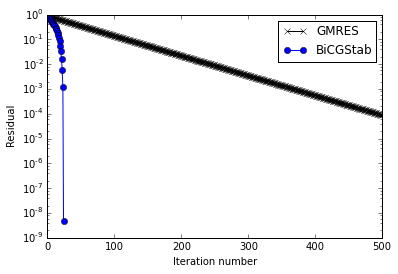# Lecture 12: Iterative methods¶

## Syllabus¶

Week 1: Matrices, vectors, matrix/vector norms, scalar products & unitary matrices
Week 2: TAs-week (Strassen, FFT, a bit of SVD)
Week 3: Matrix ranks, singular value decomposition, linear systems, eigenvalues
Week 4: Matrix decompositions: QR, LU, SVD + test + structured matrices start
Week 5: Iterative methods, preconditioners, matrix functions

## Recap of the previous lecture¶

• Test
• Short intro into sparse matrices and their storage

## Today lecture¶

Today we will talk about iterative methods, where they arise, how we store them, how we operate with them.

• Concept of iterative methods
• Richardson iteration, Chebyshev acceleration
• Jacobi/Gauss-Seidel methods
• Idea of Krylov methods
• Conjugate gradient methods for symmetric positive definite matrices
• Generalized minimal residual methods
• The Zoo of iterative methods: BiCGStab, QMR, IDR, ...
• The idea of preconditioners
• Jacobi/Gauss-Seidel as preconditioner, successive overrelaxation
• Incomplete ILU

## Direct methods¶

Iterative methods are the main tools for solving large-scale numerical linear algebra problems.

$$Ax = f,$$

If the matrix $A$ is dense, the complexity is $\mathcal{O}(N^3)$, and is feasible only up to $N \sim 10^4 - 10^5$.

If the matrix $A$ is sparse, the complexity is $\mathcal{O}(N^{\alpha})$, where $\alpha = \frac{3}{2}$ for 2D problems, and $\alpha = \frac{5}{3}$ for 3D problems (non-optimal).

But the matrix-by-vector product is computed in $\mathcal{O}(N)$ operations, can we use it?

## Matrix as a black box¶

We have now an absolutely different view on a matrix: matrix is now a linear operator, that acts on a vector,

and this action can be computed in $\mathcal{O}(N)$ operations.

This is the only information we know about the matrix: the matrix-by-vector product

Can we solve linear systems?

Of course, we can multiply by the colums of the identity matrix, and recover the full matrix, but it is not what we need.

## Richardson iteration¶

The simplest idea is the "simple iteration method" or Richardson iteration.

$$Ax = f,$$ $$\tau (Ax - f) = 0,$$ $$x + \tau (Ax - f) = x,$$ $$x_{k+1} = x_k + \tau (Ax_k - f),$$

where $\tau$ is the iteration parameter, which can be always chosen such that the method converges.

## Convergence of the Richardson method¶

Let $x_*$ be the solution; introduce an error $e_k = x_{k} - x_*$, then

$$e_{k+1} = (I + \tau A) e_k,$$

therefore if $\Vert I + \tau A \Vert < 1$ the iteration converges.

For symmetric positive definite case it is always possible to select $\tau.$

## Optimal parameter choice¶

The optimal choice for $\tau$ for $A = A^* > 0$ is (prove it!) $$\tau = \frac{2}{\lambda_{\min} + \lambda_{\max}}.$$

where $\lambda_{\min}$ is the minimal eigenvalue, and $\lambda_{\max}$ is the maximal eigenvalue of the matrix $A$.

So, to find optimal parameter, we need to know the bounds of the spectra of the matrix $A$,

and we can compute it by using power method.

## Connection to ODEs¶

The Richardson iteration has a deep connection to the Ordinary Differential Equations (ODE).

Consider a time-dependent problem

$$\frac{dy}{dt} = A y - f, \quad y(0) = y_0.$$

Then $y(t) \rightarrow A^{-1} f$ as $t \rightarrow \infty$, and the Euler scheme reads

$$\frac{(y_{k+1} - y_k)}{\tau} = A y_k - f.$$

## Convergence speed and condition number¶

Even with the optimal parameter choice, the error at the next step satisfies

$$e_{k+1} \leq e_k q, \rightarrow e_k \leq c q^k,$$

where

$$q = \frac{\lambda_{\max} - \lambda_{\min}}{\lambda_{\max} + \lambda_{\min}} = \frac{c - 1}{c+1},$$$$c = \frac{\lambda_{\max}}{\lambda_{\min}} = \mathrm{cond}(A)$$

is the condition number of $A$.

Let us do some demo...

In :
import numpy as np
import matplotlib.pyplot as plt
%matplotlib inline
import scipy as sp
import scipy.sparse
import scipy.sparse.linalg as spla
import scipy
from scipy.sparse import csc_matrix
n = 40
ex = np.ones(n);
lp1 = sp.sparse.spdiags(np.vstack((ex,  -2*ex, ex)), [-1, 0, 1], n, n, 'csr');
rhs = np.ones(n)
ev1, vec = spla.eigs(lp1, k=2, which='LR')
ev2, vec = spla.eigs(lp1, k=2, which='SR')
lam_max = ev1
lam_min = ev2

tau_opt = 2.0/(lam_max + lam_min)

fig, ax = plt.subplots()
plt.close(fig)

niters = 100
x = np.zeros(n)
res_all = []
for i in xrange(niters):
rr = lp1.dot(x) - rhs
x = x - tau_opt * rr
res_all.append(np.linalg.norm(rr))
#Convergence of an ordinary Richardson (with optimal parameter)
plt.plot(res_all)
lam_max, lam_min

Out:
((-0.0058683976325189921+0j), (-3.994131602367478+0j))## Condition number of and convergence speed¶

Thus, for ill-conditioned matrices the error of the simple iteration method decays very slowly.

This is another reason why condition number is so important:

1. It gives the bound on the error in the solution

2. It gives an estimate of the number of iterations for the iterative methods.

Main questions for the iterative method is how to make the matrix better conditioned

## Better iterative methods¶

But before preconditioners, we need to use better iterative methods.

There is a whole zoo of iterative methods, but we need to know just few of them.

## Attempt 1: Different time steps¶

Suppose we change $\tau$ every step, i.e. $$x_{k+1} = x_k + \tau_k (A x_k - f).$$

Then, $$e_{k+1} = (I - \tau_k A) e_k = (I - \tau_k A) (I - \tau_{k-1} A) e_{k-1} = \ldots = p(A) e_0,$$

where $p(A)$ is a matrix polynomial (simplest matrix function)

$$p(A) = (I - \tau_k A) \ldots (I - \tau_0 A),$$

and $p(0) = 1$.

## Optimal choice of the time steps¶

The error is written as

$$e_{k+1} = p(A) e_0,$$

where $p(0) = 1$ and $p(A)$ is a matrix polynomial.

To get better error reduction, we need to minimize

$$\Vert p(A) \Vert$$

over all possible polynomials.

## Polynomials least deviating from zeros¶

Important special case: $A = A^* > 0$.

Then $A = U \Lambda U^*$,

and

$$\Vert p(A) \Vert = \Vert U p(\Lambda) U^* \Vert = \Vert p(\Lambda) \Vert = \max_k |p(\lambda_k)| \leq \max_{a \leq \lambda \leq b} p(\lambda).$$

Thus, we need to find a polynomial such that $p(0) = 1$, that has the least possible deviation from $0$ on a given interval $[a, b]$.

## Polynomials least deviating from zeros (2)¶

We can do the affine transformation of the interval $[a, b]$ to the interval $[-1, 1]$.

The problem is then reduced to the problem of finding the polynomial least deviating from zero on an interval $[-1, 1]$

with some normalization constraint $p(c) = 1$.

## Exact solution: Chebyshev polynomials¶

The exact solution to this problem is given by the famous Chebyshev polynomials of the form

$$T_n(x) = \cos (n \arccos x)$$

## What do you need to know about Chebyshev polynomials¶

1. This is a polynomial! (we can express $T_n$ from $T_{n-1}$ and $T_{n-2}$).

2. $|T_n(x)| \leq 1$ on $x \in [-1, 1]$.

3. It has $(n+1)$ alternation points, were the the maximal absolute value is achieved (this is the sufficient and necessary condition for the optimality

4. The roots are just
$n \arccos x_k = \frac{\pi}{2} + \pi k, \rightarrow x_k = \cos \frac{\pi(k + 0.5)}{n}$

We can plot them...

In :
import numpy as np
import matplotlib.pyplot as plt
plt.xkcd()
%matplotlib inline
x = np.linspace(-1, 2, 128)
p = np.polynomial.Chebyshev((0, 0, 0, 0, 0, 0, 0, 0, 0, 1), (-1, 1)) #These are Chebyshev series, a proto of "chebfun system" in MATLAB
plt.plot(x, p(x))

Out:
[<matplotlib.lines.Line2D at 0x11245f5d0>]## Convergence of the Chebyshev-accelerated Richardson iteration¶

Given the roots of the polynomials $x_k$, the best parameters are determined as

$$\tau_k = \frac{1}{x_k}.$$

The convergence (we only give the result without the proof) is now given by

$$e_{k+1} \leq c q^k, \quad q = \frac{\sqrt{c}-1}{\sqrt{c}+1},$$

where $c = \mathrm{cond}(A)$.

## Beyond Chebyshev¶

We have made an important assumption about the spectra: it is contained within an interval over the real line (and we need to know the bounds)

If the spectra is contained within two intervals, and we know the bounds, we can also put the optimization problem

for the optimal polynomial.

## Spectra of the matrix contain on multiple segments¶

For the case of two segments the best polynomial is given by Zolotarev polynomials (expressed in terms of elliptic functions)

For the case of more than two segments the best polynomial can be expressed in terms of hyperelliptic functions## How can we make it better¶

The implementation of the Chebyshev acceleration requires the knowledge of the spectra.

It only stores the previous vector $x_k$ and computes the new correction vector

$$r_k = A x_k - f.$$

It belongs to the class of two-term iterative methods.

It appears that if we abandon this and store more vectors, then we can go without the spectra estimation (and better convergence in practice)!

## Crucial point¶

The Chebyshev method produces the approximation of the form

$$x_{k+1} = p(A) f,$$

i.e. it lies in the the Krylov subspace of the matrix which is defined as

$$K(A, f) = \mathrm{Span}(f, Af, A^2 f, \ldots, )$$

The most natural approach then is to find the vector in this linear subspace that minimizes

certain norm of the error

## What to minimize in the Krylov subspace¶

The ideal way is to minimize

$$\Vert x_k - x_* \Vert,$$

over the Krylov subspace $K_n(A, f)$

where $x_*$ is the true solution, but we do not know it!

Then we can minimize the residual:

$$\Vert f - Ax \Vert,$$

For the SPD case we can do better!

## Symmetric positive definite case¶

Consider again $A = A^* > 0$

Then we can introduce A-norm as

$$\Vert x \Vert_A = \sqrt{(Ax, x)}.$$

Let us verify that it is the norm (on the whiteboard).

The most popular iterative method today (for positive definite matrices) is the conjugate gradient method:

It minimizes the A-norm of the error over the Krylov subspace

$$x_{k+1} = \arg_{x \in K_n(A, f)} \min \Vert x - x_* \Vert_A$$

And we can compute the minimization without knowing the error!

## Why we can compute A-norm of the error¶

Given $x$ we can compute the A-norm of the error:

$$\Vert x - x_* \Vert_A^2 = (A (x - x_*), (x - x_*))$$

## How to get the CG formulas¶

Consider the one step:

$x_i = x_0 + y_i, \quad y_i = \arg \min \Vert x_0 + y - z \Vert_A, \quad y \in K_n$.

Using Pythagoreus theorem,

$(x_n, y) = (z, y)_A$ for all $y \in K_n$, $\rightarrow$ $r_n = Ax_n - f \perp K_n$

After some machinery...

## CG-method: formulas¶

We get the following three-term recurrence relations for the CG method: $$\alpha_n = (r_{n-1}, r_{n-1})/(Ap_n, p_n),$$ $$x_n = x_{n-1} + \alpha_n p_n,$$ $$r_n = r_{n-1} - \alpha_n A p_n,$$ $$\beta_n = (r_n, r_n)/(r_{n-1},r_{n-1}),$$ $$p_{n+1} = r_n + \beta_n p_n$$

The vectors $p_i$ constitute an A-orthogonal basis in $K_n$.

We store $x$, $p$, $r$ (three vectors).

## CG-method: history¶

CG-method has a long history:

1. In was proposed in by Hestenes and Stiefel in 1952.
2. In exact arithmetics it should give exact solution at $n$ iterations
3. In floating-point arithmetics it did not - people thought it is unstable compared to Gaussian elimination
4. Only decades later it was realized that it is a wonderful iterative method

## CG-method: properties¶

1. Convergence estimate (same convergence speed as Chebyshev) $$\Vert e_k \Vert \leq 2 \Big( \frac{\sqrt{c} - 1}{\sqrt{c} + 1} \Big)^k \Vert e_0 \Vert.$$

2. CG mystery (why it is much better than Chebyshev) is clustering of eigenvalues:

if there are $k$ "outliers", then in $k$ iterations they are "removed" (we can illustrate it on the board)

## Non-symmetric matrices¶

CG only works for SPD matrices, and (sometimes) for symmetric matrices (although we can divide by zero).

It completely does not work for the non-symmetric matrices.

For non-symmetric matrices the two main methods are

1. GMRES (Generalized minimal residual method)

## GMRES¶

GMRES idea is very simple: we construct orthogonal basis in the Krylov subspace.

Given the basis $q_1, \ldots, q_n$ we compute the residual $r_ n = Ax_n - f$ and orthogonalize it to the basis.

A Python realization is available as scipy.sparse.linalg.gmres (although quite buggy).

The main disadvantage of GMRES: we have to store all the vectors, so the memory costs grows with each step.

We can do restarts (i.e. get a new residual and a new Krylov subspace): we find some approximate solution $x$

and now solve the linear system for the correction:

$$A(x + e) = f, \quad Ae = f - Ax,$$

and generate the new Krylov subspace.

BiCGStab method avoids that using "short recurrences" like in the CG method

## Idea of BiCGStab¶

Idea of BiCG method is to use the normal equations:

$$A^* A x = A^* f,$$

and apply the CG method to it.

The condition number has squared, thus we need stabilization.

The stabilization idea proposed by Van der Vorst et al. gives the most practically used iterative method for non-symmetric systems.

Let us do some demo

In :
#### import numpy as np
import scipy.sparse.linalg
%matplotlib inline
import matplotlib.pyplot as plt
import numpy as np
import scipy as sp
n = 50
ex = np.ones(n);
lp1 = -sp.sparse.spdiags(np.vstack((ex,  -2*ex, ex)), [-1, 0, 1], n, n, 'csr');
rhs = np.ones(n)
ee = sp.sparse.eye(n)

#lp2 = sp.kron(lp1, ee) + sp.kron(ee, lp1)
#rhs = np.ones(n * n)
res_all = []
res_all_bicg = []
def my_print(r):
res_all.append(r)

def my_print2(x): #For BiCGStab they have another callback, please rewrite
res_all_bicg.append(np.linalg.norm(lp1.dot(x) - rhs))
#res_all_bicg = np.array(res_all_bicg)
sol = scipy.sparse.linalg.gmres(lp1, rhs, restart=10, callback=my_print)
plt.semilogy(res_all, marker='x',color='k', label='GMRES')
sol2 = scipy.sparse.linalg.bicgstab(lp1, rhs, x0=np.zeros(n), callback=my_print2)
res_all_bicg = np.array(res_all_bicg)/res_all_bicg

plt.xlabel('Iteration number')
plt.ylabel('Residual')
plt.semilogy(res_all_bicg, label='BiCGStab', marker='o')
plt.legend(loc='best')

Out:
<matplotlib.legend.Legend at 0x113611d50>## Battling the condition number¶

The condition number problem is un-avoidable if only the matrix-by-vector product is used.

Thus we need an army of preconditioners to solve it.

There are several general purpose preconditioners that we can use (short list today, more details tomorrow),

but often for a particular problem a special design is needed.

## Preconditioner: general concept¶

The general concept of the preconditioner is simple:

Given a linear system

$$A x = f,$$

we want to find the matrix $P$ such that

1. We can easily solve $Py = g$ for any $g$
2. Condition number of $AP^{-1}$ is better

Then we solve for (right preconditioner)

$$AP^{-1} y = f.$$

or (left preconditioner)

$$P^{-1} A y = f,$$

The best choice of course is $P = A,$ but this does not make life easier.

## Other iterative methods as preconditioners¶

There are other iterative methods that we have not mentioned.

1. Jacobi method
2. Gauss-Seidel method
3. SSOR (Successive over-relaxation)

## Jacobi method¶

Jacobi method is when you express the diagonal element:

$$a_{ii} x_i = -\sum_{i \ne j} a_{ij} x_j + f_i$$

and use this to iteratively update $x_i$.

From the matrix viewpoint we use

$$D = \mathrm{diag}(A).$$

## Gauss-Seidel (as preconditioner)¶

Another well-known method is Gauss-Seidel method. Given $A = A^{*} > 0$ we have

$$A = L + D + L^{*},$$

where $D$ is the diagonal of $A$, $L$ is lower-triangular part, and Gauss-Seidel is $(L + D)^{-1}$.

Good news: $\rho(I + (L+D)^{-1} A) < 1,$

where $\rho$ is the spectral radius.

## Successive overrelaxation (as preconditioner)¶

We can even introduce a parameter into the preconditioner, giving a SSOR method:

$$(D + w L) x = w b - (w U + (w-1) D) x,$$$$P = (D+wL)^{-1} (w U + (w-1) D).$$

Optimal selection of $w$ is not trivial.

## Others (but very important, see it tomorrow)¶

• Incomplete LU for sparse matrices (tomorrow)
• Algebraic multigrid
• Sparse approximate inverse
• Domain decomposition

## Take home message¶

• Iterative methods are the core
• Krylov subspaces are the core
• Condition number is the problem
• Conjugate gradient is the best for SPD
• GMRES/BiCGStab are the best for non-symmetric
• Preconditioners are crucial (and often do not work)

## Next lecture¶

• More on preconditioners for sparse matrices

# Questions?¶

In :
from IPython.core.display import HTML
def css_styling():